### Home > A2C > Chapter 9 > Lesson 9.1.2 > Problem9-41

9-41.
1. What is the degree of each polynomial function below? Homework Help ✎

1. P(x) = 0.08x2 + 28x

2.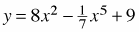3. f (x) = 5(x + 3)(x − 2)(x + 7)

4. y = (x − 3)2 (x + 1)(x3 + 1)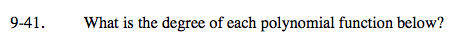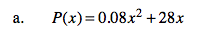Look for the highest-valued exponent.

2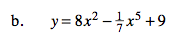See part (a).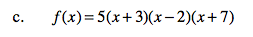See part (a). Simplify first.

3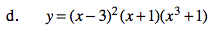See part (c).